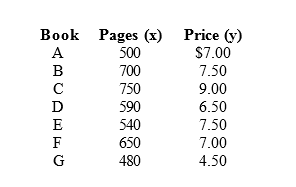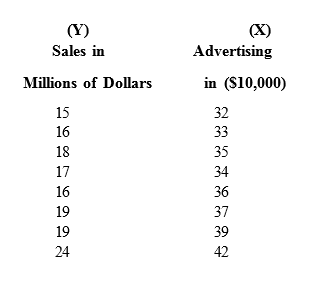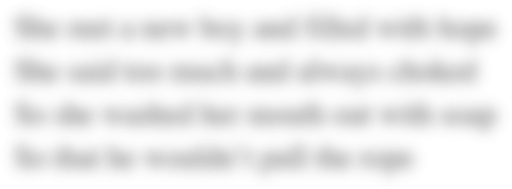# BA215 W7 Written Assignment Week 7 Assignment

• Question 1

Assume you have noted the following prices for paperback books and the number of pages that each book contains.
Develop a least-squares estimated regression line.1. Compute the coefficient of determination and explain its meaning.
2. Compute the correlation coefficient between the price and the number of pages. Test to see if x and y are related. Use α = 0.10.

Question 2

The following data represent a company's yearly sales volume and its advertising expenditure over a period of 8 years.1. Develop a scatter diagram of sales versus advertising and explain what it shows regarding the relationship between sales and advertising.
2. Use the method of least squares to compute an estimated regression line between sales and advertising.
3. If the company's advertising expenditure is \$400,000, what are the predicted sales? Give the answer in dollars.
4. What does the slope of the estimated regression line indicate?

Field of study:
Date Due:
Tuesday, September 25, 2018